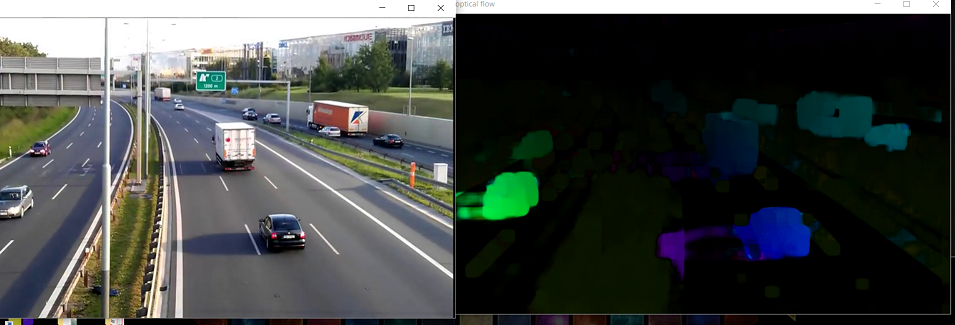# Python OpenCV – Dense optical flow

Prerequisites: Python OpenCV, Grayscaling

Optical flow is the motion of objects between the consecutive frames of the sequence, caused by the relative motion between the camera and the object. It can be of two types-Sparse Optical flow and Dense Optical flow.

## Dense Optical flow

Dense Optical flow computes the optical flow vector for every pixel of the frame which may be responsible for its slow speed but leading to a better accurate result. It can be used for detecting motion in the videos, video segmentation, learning structure from motion. There can be various kinds of implementations of dense optical flow. The example below will follow the Farneback method along with OpenCV.

## Franeback Method

The first step is that the method approximates the windows of image frames by a quadratic polynomial with the help of the polynomial expansion transform. Next, by observing how the polynomial transforms under the state of motion. i.e. to estimate displacement fields. Dense optical flow is computed, after a series of refinements.

For OpenCV’s implementation, the magnitude and direction of optical flow from a 2-D channel array of flow vectors are computed for the optical flow problem. The angle (direction) of flow by hue is visualized and the distance (magnitude) of flow by the value of HSV color representation. The strength of HSV is always set to a maximum of 255 for optimal visibility.
The method defined is caclopticalFlowFarneback() .

Below is the implementation.

 `import` `cv2 as cv ` `import` `numpy as np ` ` `  ` `  `# The video feed is read in as ` `# a VideoCapture object ` `cap ``=` `cv.VideoCapture(``"videoplayback.mp4"``) ` ` `  `# ret = a boolean return value from ` `# getting the frame, first_frame = the ` `# first frame in the entire video sequence ` `ret, first_frame ``=` `cap.read() ` ` `  `# Converts frame to grayscale because we ` `# only need the luminance channel for ` `# detecting edges - less computationally  ` `# expensive ` `prev_gray ``=` `cv.cvtColor(first_frame, cv.COLOR_BGR2GRAY) ` ` `  `# Creates an image filled with zero ` `# intensities with the same dimensions  ` `# as the frame ` `mask ``=` `np.zeros_like(first_frame) ` ` `  `# Sets image saturation to maximum ` `mask[..., ``1``] ``=` `255` ` `  `while``(cap.isOpened()): ` `     `  `    ``# ret = a boolean return value from getting ` `    ``# the frame, frame = the current frame being ` `    ``# projected in the video ` `    ``ret, frame ``=` `cap.read() ` `     `  `    ``# Opens a new window and displays the input ` `    ``# frame ` `    ``cv.imshow(``"input"``, frame) ` `     `  `    ``# Converts each frame to grayscale - we previously  ` `    ``# only converted the first frame to grayscale ` `    ``gray ``=` `cv.cvtColor(frame, cv.COLOR_BGR2GRAY) ` `     `  `    ``# Calculates dense optical flow by Farneback method ` `    ``flow ``=` `cv.calcOpticalFlowFarneback(prev_gray, gray,  ` `                                       ``None``, ` `                                       ``0.5``, ``3``, ``15``, ``3``, ``5``, ``1.2``, ``0``) ` `     `  `    ``# Computes the magnitude and angle of the 2D vectors ` `    ``magnitude, angle ``=` `cv.cartToPolar(flow[..., ``0``], flow[..., ``1``]) ` `     `  `    ``# Sets image hue according to the optical flow  ` `    ``# direction ` `    ``mask[..., ``0``] ``=` `angle ``*` `180` `/` `np.pi ``/` `2` `     `  `    ``# Sets image value according to the optical flow ` `    ``# magnitude (normalized) ` `    ``mask[..., ``2``] ``=` `cv.normalize(magnitude, ``None``, ``0``, ``255``, cv.NORM_MINMAX) ` `     `  `    ``# Converts HSV to RGB (BGR) color representation ` `    ``rgb ``=` `cv.cvtColor(mask, cv.COLOR_HSV2BGR) ` `     `  `    ``# Opens a new window and displays the output frame ` `    ``cv.imshow(``"dense optical flow"``, rgb) ` `     `  `    ``# Updates previous frame ` `    ``prev_gray ``=` `gray ` `     `  `    ``# Frames are read by intervals of 1 millisecond. The ` `    ``# programs breaks out of the while loop when the ` `    ``# user presses the 'q' key ` `    ``if` `cv.waitKey(``1``) & ``0xFF` `=``=` `ord``(``'q'``): ` `        ``break` ` `  `# The following frees up resources and ` `# closes all windows ` `cap.release() ` `cv.destroyAllWindows() `

OutputWhether you're preparing for your first job interview or aiming to upskill in this ever-evolving tech landscape, GeeksforGeeks Courses are your key to success. We provide top-quality content at affordable prices, all geared towards accelerating your growth in a time-bound manner. Join the millions we've already empowered, and we're here to do the same for you. Don't miss out - check it out now!

Previous
Next